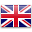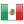Dr. Alex Jimenez, El Paso's Chiropractor
I hope you have enjoyed our blog posts on various health, nutritional and injury related topics. Please don't hesitate in calling us or myself if you have questions when the need to seek care arises. Call the office or myself. Office 915-850-0900 - Cell 915-540-8444 Great Regards. Dr. J

Bioimpedance Analysis (BIA) is defined as the analysis of resistance and reactance in the human body.

• Energy dissipation is called resistance (R).
• Energy storage is called reactance (X).
• Units of resistance and reactance are called ohms.
• Resistance and reactance taken together create impedance (Z).
• Fat-free mass in the human body is proportional to its resistance.
• Body cell mass is proportional to its reactance.
• A bioimpedance analyzer is a device that contains a highly precise frequency meter, a processor, and a printer. The meter is called an impedance meter. It measures resistance and reactance.
• The microprocessor computes the size of the fat-free mass and body cell mass along with all subsequent calculations.
• A bioimpedance analyzer measures resistance and reactance, computes fat-free mass, body cell mass, total body water, and intracellular water.
• To measure resistance and reactance, the bioimpedance analyzer generates a small electrical current to the body.

### Measurements

• Bioimpedance analysis is an assessment of change in electrical tissue conductivity, which indicates altered body composition.
• The following electrical characteristics are measured and used to evaluate body composition.
• Impedance (Z)
• Impedance is a measurement of a medium to conduct current. It is a ratio of voltage injected current in a conductive medium and has two components: resistance and reactance.
• Resistance (R)
• Resistance is the component of impedance related to the dissipation of energy.
• Reactance (X)
• Reactance is the component of impedance related to the storage of energy.
• Phase Angle (α)
• Phase angle is a time delay between stimulating current and the voltage generated by an alternating current. The phase angle is expressed in degrees.
• Electrical characteristics and their relation to each other?
• The time relationship of voltage, current, and phase is shown below.

### The Circuit

• Water is the body’s conductor and determines the resistance.
• Electrolytic fluid in fat-free mass consists of water and charged ions that conduct electrical current.
• Extracellular fluid (water and ionized sodium Na+) provides a low-resistance pathway.
• Intracellular fluid (water and ionized potassium K+) provides a low-resistance pathway.
• Fat mass is non-conductive because there is no water.
• Cell membranes within intracellular mass determine reactance.
• The cell membranes consist of a layer of nonconductive, lipophilic material interposed between two layers of conductive molecules. They are tiny capacitors that store electrical charge.
• Extracellular pathway (which is sodium ionized water) is represented by single resistance.
• Intracellular pathway (potassium ionized water and cell membranes) is represented by resistance and reactance.
• Total resistance is proportional to the amount of fat-free mass.
• Reactance is proportional to body cell mass (intracellular mass).
• The meter reports total resistance and total reactance to the microprocessor.

### Equation for Regression

• Bioimpedance analyzers use algorithms to compute amounts of fat-free mass, body cell mass, body water, and intracellular water.
• What is the regression equation?
• This equation is a mathematical formula that predicts the relationship between two or more variables based on the analysis of data.
• For example, total body water regression equation
• (TBW) = form:
• TBW = a * Ht2 / R + b * Weight + c * Age + d
• The terms are Ht2 / R, Weight, and Age.
• The weighting constants are a, b, c, and d.
• Resistance, height, weight, gender, and age are supplied for each subject. A computer then generates an algorithm which best describes the relationship between TBW, resistance, height, weight, gender, and age.
• This is called Regression Analysis.

#### How do you use regression equations?

• Once the bioimpedance analyzer measures the resistance and reactance, the results are sent to the microprocessor to perform the calculations. All the equations, (FFM, BCM, TBW, and ICW) are encoded into the analyzer’s software.

### Diagram

• Fat-Free Mass
• Fat-free mass is derived by applying a small alternating current to the body.
• The resistance of the body is measured using the measured resistance (R), the analyzer estimates the fat-free mass.
• Body Cell Mass
• Body cell mass is derived by measuring both the resistance (R) and reactance (X) of the body. With this measurement, the analyzer estimates body cell mass.
• Total Body Water
• Using the measured resistance, the analyzer estimates total body water.
• Intracellular Water
• Using the measured resistance and reactance, the analyzer estimates intracellular water.

Clinic Location 1

Address: 11860 Vista Del Sol Dr Suite 128
El Paso, TX 79936
Phone: (915) 412-6677
Email: Send Email
Web: www.DrAlexJimenez.com

Clinic Location 2

Address: 6440 Gateway East, Building B
El Paso, TX 79905
Phone: (915) 850-0900
Email: Send Email
Web: www.ElPasoBackClinic.com

Clinic Location 3

Address: 1700 N Zaragoza Rd # 117
El Paso, TX 79936
Phone: (915) 850-0900
Email: Send Email
Web: www.ChiropracticScientist.com

Push As Rx Crossfit & Rehab

Address: 6440 Gateway East, Building B
El Paso, TX 79905
Phone: (915) 412-6677
Email: Send Email
Web: www.PushAsRx.com

El Paso, TX 79902
Phone: (915) 412-6677
Email: Send Email
Web: www.PushAsRx.com

1. Online Appointments or Consultations:  https://bit.ly/Book-Online-Appointment
2. Online Physical Injury / Accident Intake Form:
3. Online Functional Medicine Assessment: https://bit.ly/functionmed

DisclaimerENES